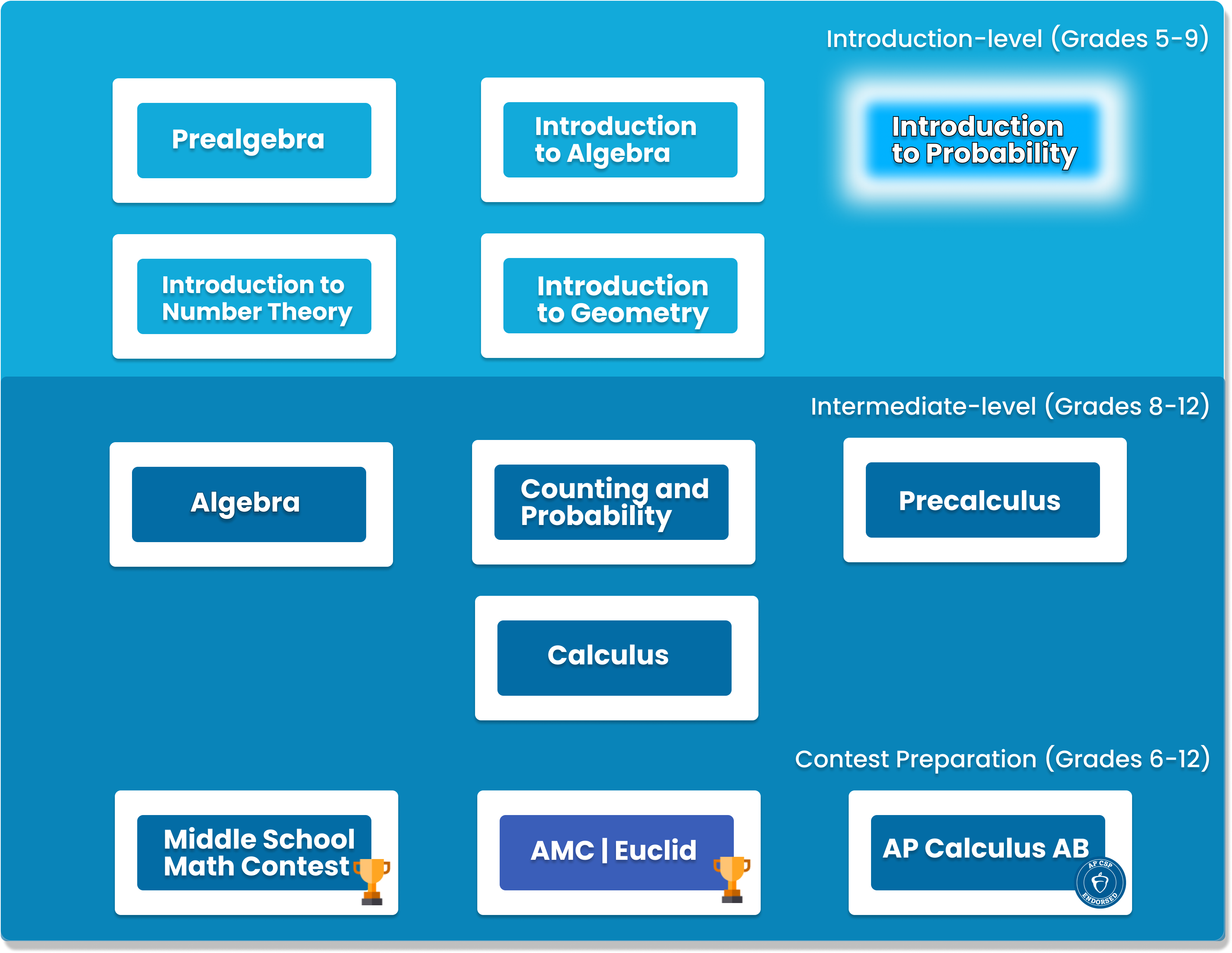# Introduction to Counting and Probability

This class will teach students in grades 7-10 the foundations of counting and probability. Students will learn a variety of topics that expand beyond the regular school curriculum. Students will finish the course with an in-depth knowledge of permutations; combinations; probability; Pascal’s Triangle and the Binomial Theorem.##### Who is this course for
This Course is for Grade 5-Grade 9 Students who want to advance their knowledge in counting and probability.
This course typically takes 3-6 months to complete. This depends on the student's experience and how fast they can master the concepts and the knowledge. Our instructors move at the pace of the student, it may take extra time for some students to reinforce what they have learned.
##### Curriculum
###### Chapter 1: Counting is ArithmeticCounting by addition and multiplication, permutations
###### Chapter 2: Basic Counting TechniquesCount by cases, Complementary counting, constructive counting, counting with restrictions
###### Chapter 3: Correcting for OvercountingPermutations with repeated elements, counting pairs and with symmetry
###### Chapter 4: Committees and CombinationsCombinations
###### Chapter 5: More with CombinationsCounting Paths on a grid, number of ways to form committee, distinguishability
###### Chapter 6: Some Harder Counting Problems###### Chapter 7: Introduction to ProbabilityProbability of equally likely outcomes, applications with counting techniques
###### Chapter 8: Basic Probability TechniquesComplementary probabilities, dependent event
###### Chapter 9: Think about it!Learn faster approach to solve probability problems
###### Chapter 10: Geometric probabilityProbability involving lengths and areas
###### Chapter 11: Expected ValueDefinition and properties of expected value
###### Chapter 12: Pascal’s TriangleConstruction of Pascal’s triangle, relationship with combinatorics
###### Chapter 13: The hockey stick identityDerivation of the hockey stick identity, and its application
###### Chapter 14: Binomial TheoremDerivation and application of the binomial theorem
###### Chapter 15: More Challenging ProblemsHard problems in counting that use combinations of topics above

### You Might Also Be Interested In Our Elective Courses##### Python Level 1
AGES 10 - 15

Designed for beginners, this level is designed to teach the basic fundamentals and design principles of Python, with the help of Turtle graphics and PyGame. This includes: variables, conditional statements, loop basics, and functions.

These concepts are transferable to any other programming language. Throughout the way, students will create projects in order to apply the concepts they have learned, and to solidify their knowledge.##### Python Level 2
AGES 10 - 15

Level 2 dives deeper into the basics of Python for a more thorough understanding and introduces advanced topics. Students will build on knowledge from Level 1 and work with data structures, advanced loops, algorithms, and object-oriented programming, and create games based on what they learn.

Students will complete the course with a solid understanding of Python fundamentals.##### Java Level 1
AGES 10 - 15

This beginner-friendly course serves as an introduction to the Java programming language. Students will learn the fundamentals of Java along with core computer science concepts. Concepts covered in this course include Java syntax, types and identifiers, operators, if statements, loops, arrays and more.

Students will apply these concepts to create fun games such as Tic-Tac-Toe.